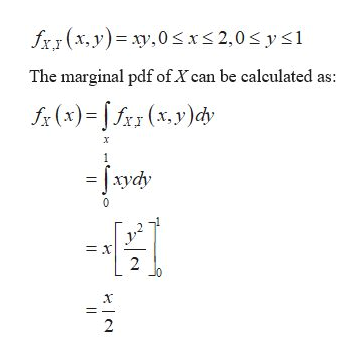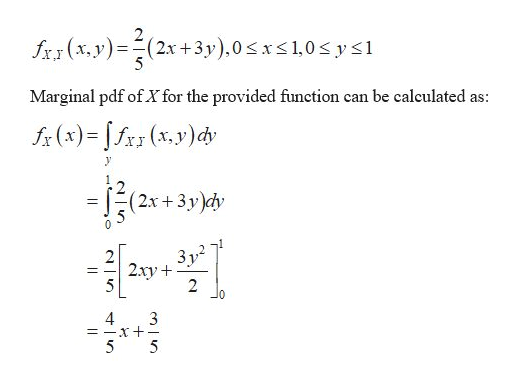# Find fX(x) and fY (y) for each of the following joint pdfs.(a) fX,Y (x, y) = xy, 0 ≤ x ≤ 2, 0 ≤ y ≤ 1.(b) fX,Y (x, y) = 2/5 (2x + 3y), 0 ≤ x ≤ 1, 0 ≤ y ≤ 1.

Question
63 views

Find fX(x) and fY (y) for each of the following joint pdfs.

(a) fX,Y (x, y) = xy, 0 ≤ x ≤ 2, 0 ≤ y ≤ 1.

(b) fX,Y (x, y) = 2/5 (2x + 3y), 0 ≤ x ≤ 1, 0 ≤ y ≤ 1.

check_circle

Step 1

(a)

Provided joint pdf of (X, Y) ishelp_outlineImage Transcriptionclosefxy (x,y) ,0x< 2,0 < y <1 The marginal pdf of X can be calculated as: fx(x)=fx(x.y)dy x = x 2 2 fullscreen
Step 2

Similarly, marginal pdf of Y can be calculated as:

Step 3

(b)

Provided joint pdf of (X, ...help_outlineImage Transcriptionclose2 (2.x+3y),0 sx1,0 y s1 .y)= fxy(x,y Marginal pdf of X for the provided function can be calculated as: (x) (xy)c (2x+3y)cy 3y2 2xy 2 4 3 5 fullscreen

### Want to see the full answer?

See Solution

#### Want to see this answer and more?

Solutions are written by subject experts who are available 24/7. Questions are typically answered within 1 hour.*

See Solution
*Response times may vary by subject and question.
Tagged in

### Other